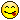0
|
7479 Views
|
4 Replies
|
0 Total Likes
View groups...
Share
GROUPS:

# Second derivative from Mathematica is wrong!

Posted 10 years ago
 Hellow everyone,I have this very strange phenomenon happening on my computer. When I try to calculate a simple ODEDSolve[{y''[x] == y[x]}, y[x], x]It outputsDSolve[{True}, y[x], x]with an error message Equation or list of equations expected instead of True in the first argument {True}I looked deeper and I found the following. If I doD[y[x], {x, 1}]I get Derivative[y][x], which is correct.But if I doD[y[x], {x, 2}]I gety[x]And if I doD[y[x], {x, 3}]I get Derivative[y][x]again! It shows that whenever I do a second derivative on my Mathematica, it always gives me the original function back. Am I doing something wrong here? Or is there anything I can fix this problem?Thanks for reading.Tom
4 Replies
Sort By:
Posted 10 years ago
 Simon, I believe I figured out why that happened. In my first attempt to use the DSolve routine, I mistakenly used '=' for '=='. That assignment side effect ruin all later computations.Thank you guys. Now the expressions work perfectly! I am still new to the language so sorry for the sillinessBest regards,Tom
Posted 10 years ago
 Restart Mathematica, type exactly and only this into a notebook, nothing more, nothing lessDSolve[{y''[x] == y[x]}, y[x], x]followed by shift and enter and I get this{{y[x] -> E^x C + E^-x C}}I suspect the reason for what you saw was that you had previously somehow assigned values to some variables or functions. Mathematica does not make that obvious and the results can be very confusing. Sometimes restarting will resolve this. Sometimes using the menu to quit the kernel will resolve this.
Posted 10 years ago
 Looks like you've accidentaly assigned a value to Derivative: (* How the y''[x] expression looks without nice syntax: *) y''[x]//FullForm (* Derivative[y][x] *)  DSolve[{y''[x] == y[x]}, y[x], x] (* {{y[x] -> E^x C + E^-x C}} *)  y''[x] = whoops D[y[x], {x, 2}](* whoops *)whoops = y[x]D[y[x], {x,2}](* y[x] *)(* Let's try to fix it by clearing y *)ClearAll[y];D[y[x], {x,2}](* y[x] didn't work *)(* Clear the strange definition by: *)y''[x]=.D[y[x], {x,2}](* y''[x] *)What goes wrong is the assignment to y'' which is easier to understand by remembering the FullForm:Derivative[y][x] = somethingmaking all kinds of strange things happen.
Posted 10 years ago
 By the way, I am using a student version of Mathematica 9. Version number 9.0.1.0, on a 64bit Linux machine.